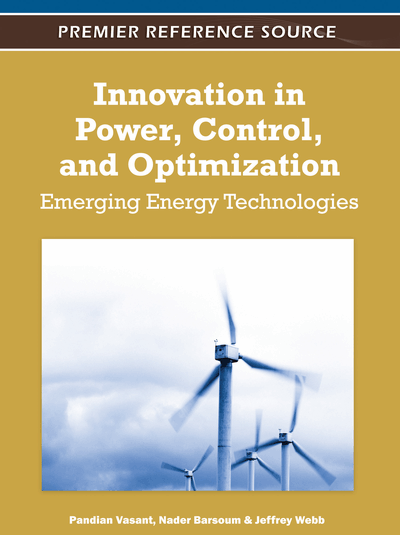# Hopfield Lagrange Network for Economic Load Dispatch

Vo Ngoc Dieu (Asian Institute of Technology, Thailand) and Weerakorn Ongsakul (Asian Institute of Technology, Thailand)
DOI: 10.4018/978-1-61350-138-2.ch002

## Abstract

In this chapter, a Hopfield Lagrange network (HLN) is proposed for solving economic load dispatch (ELD) problems. HLN is a combination of Lagrangian function and continuous Hopfield neural network where the Lagrangian function is directly used as the energy function for the continuous Hopfield neural network. In the HLN method, its energy function augmented by Hopfield terms from the continuous Hopfield network could damp out oscillation of the conventional Hopfield network during the convergence process. Consequently, the proposed HLN can overcome the disadvantages of the conventional Hopfield network in solving optimization problems for its simpler implementation, better global solution, faster convergence time, and larger scale applications. The proposed method has been tested on different ELD problems including all thermal units, thermal units with fuel constraint, and both thermal and hydro units. The obtained results from the test cases have shown that the proposed method is effective and efficient for solving the ELD problems. Therefore, the HLN method is the new contribution to the development of new methods for solving optimization problems in power systems.
Chapter Preview
Top

## Introduction

Power system engineering has the longest history of development among the various areas within electrical engineering. Since the practical numerical optimization methods applied to power system engineering and operation, they have been playing a very important role in economic operation of power systems. The value contributed by the power system optimization is considerable in economics for large utilities from fuel cost, operational reliability, and security.

In power system operation, the problem allocating among the available thermal power generating units to the customers’ load demands in an economic, secure and reliable way has been identified and received great attention since the beginning of the 20th century (Happ, 1977; Chowdhury & Rahman, 1990). The problem has been formulated as an optimization problem which is to minimize fuel cost of overall online generating units while satisfying load demand and other constraints over a considered schedule time. This problem has been frequently known as the economic load dispatch (ELD) problem. The simplest form of the ELD problem can be considered as to minimize the total fuel cost through determining the allocation of power generation of each units among a set of committed units in a thermal power plant subject to a constraint that total power generation equals to load demand or among a set of committed units in different thermal power plants subject to a constraint that total power generation equals to load demand plus transmission power loss. This type of ELD problem is referred to the conventional problem applied in vertically integrated power systems. The more complicated ELD problems can be arranged in ascending order as more constraints added:

• The generator capacity limits are considered.

• There are conflicts among the considerations such as economy, security, and emission.

• Fuel, labor and maintenances constraints are added.

• The optimal power solution considers both active and reactive control variables.

• The problem is considered in deregulated environment.

In fact, the ELD problem involves the solution of two different problems. The first one is pre-dispatch problem or unit commitment (UC) problem which requires selecting optimal units among the available ones to meet the expected load demand with a specified reserve of operation over a scheduled time horizon. The last one is the on-line ELD which requires satisfying the load demand among the actually committed units so as to minimize total cost of supplying the requirement of the system.

Due to the great values contributed to the economic operation of power systems, the ELD problems have been attracted the attention many researchers from 1920s with several solution methods proposed to deal with more complicated and larger scale problems. One of the earliest methods to find economic results for power generation schedule was known as equal incremental method, where the optimum solution is obtained when all the marginal cost of the committed generating units are equal (Stahl, 1930, 1931). Since digital computers were used in 1950s the numerical methods have been continuously developed for solving more complicated and larger problems with more efficient and better quality solution. Several solution methods have been proposed for solving the problems including conventional, artificial intelligent, and hybrid methods. Many conventional methods have been widely used for solving the ELD problems such as dynamic programming (DP) (Liang, Glover & Glover, 1992), linear programming (LP) (Wells, 1968), quadratic programming (QP) (Irving & Sterling, 1985), interior point method (IPM) (Ponnambalam, Quintana & Vanelli, 1992), and Lagrangian relaxation (LR) (Fisher, 1973). Conventional methods require the models of optimal generation scheduling problems to be represented as piecewise linear or polynomial approximations of monotonically increasing nature. However, such an approximation may lead to sub-optimal solution, resulting in huge loss of revenue over the time. Hence, the new trend in the recent time is to use more realistic models of hydro and thermal plants.

## Complete Chapter List

Search this Book:
Reset